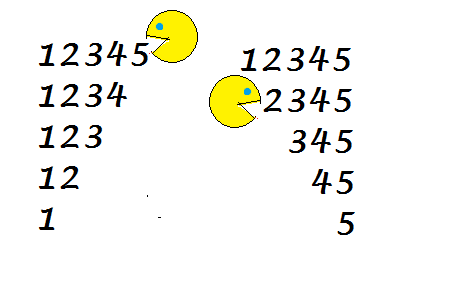# Eating the tasty numbers!Given a positive integer $(1,2,3,\ldots$), we start $\color{#3D99F6}{\text{eating the number}}$ from either left or right i.e. we start removing its digits one by one from left to right, or from right to left.

We define a set $\color{#3D99F6}{\text{dish}}$ of the number, which is obtained by noting the number formed after eating every digit (The original number is also included) .

The $\color{#3D99F6}{\text{taste}}$ of a number is sum of all numbers in that number's dish.

What is the smallest non-palindromic number which when eaten from left gives same taste as eating from right?

Details and Assumptions:

• The dish of a number can be obtained in 2 ways, either eating from left or eating from right and hence there'll be 2 tastes for each number (maybe the same, that's where you count the number!)

• Example of a dish, dish of the number 12635 as eaten from left will be $\{12635,2635,635,35,5\}$ and its dish when eaten from right will be $\{12635,1263,126,12,1\}$

• Taste of the number 123 will be $123+23+3 = 149$ from left and it will be $123+12+1 = 136$ from right.

• A non-palindromic number is the one which is not the same when read from left or from right, e.g. $12321 , 22 , 1441, 8$ are some examples of palindromic numbers, whereas $98,234,239478$ are some non-palindromic numbers.

×